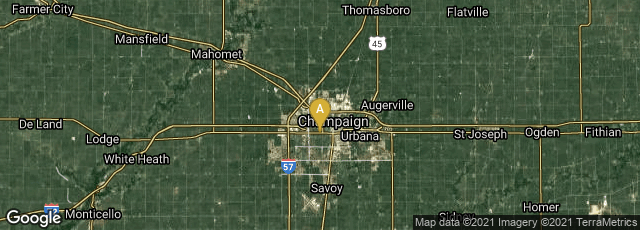A: Champaign, Illinois, United States

# Mathematica 1.0

1988

In 1988 physicist and mathematician Stephen Wolfram and Wolfram Research, Champaign, Illinois, introduced Mathematica 1.0, "a computational software program used in scientific, engineering, and mathematical fields and other areas of technical computing" with powerful two dimensional and three dimensional visualization tools.

Mathematica evolved from Symbolic Manipulation Program, usually called SMP, "a computer algebra system designed by Chris A. Cole and Stephen Wolfram at Caltech circa 1979, and initially developed in the Caltech physics department under Wolfram's leadership .... SMP was essentially Version Zero of the more ambitious Mathematica system.

"SMP was influenced by the earlier computer algebra systems Macsyma (of which Wolfram was a user) and Schoonschip (whose code Wolfram studied)" (Wikipedia article on Symbolic Manipulation Program, accessed 05-16-2009).